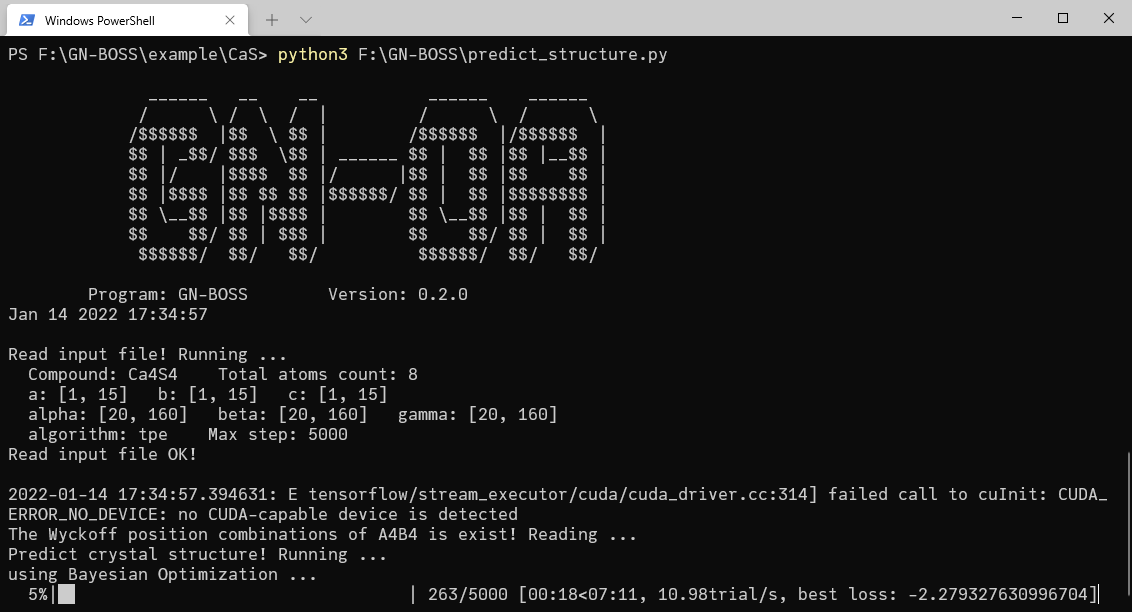# GN-OA

GN-OA is a crystal structure prediction tool, which can predict crystal structures from scratch with extremely low computational cost.

In GN-OA a graph network (GN) is adopted to establish a correlation model between the crystal structure and formation enthalpies, and optimization algorithm (OA) is used to accelerate the search for crystal structure with optimal formation enthalpy. The approach of combining GN and OA for crystal structure searching (GN-OA) can predict crystal structures at given chemical compositions with additional constraints on cell shapes and lattice symmetries.

## System requirements

• Python >= 3.6
• pymatgen >= 2020.8.3
• tensorflow >= 2.3.0
• megnet >= 1.1.8
• hyperopt >= 0.2.4
• sko(scikit-opt) >= 0.6.1

## Getting started

#### 1. Training the GN model

• (1) Data preparation

• a) MatBench dataset

See the file ./data/matbench_dataset.py

• b) OQMD

• (2) Training the GN model

We provided the origin MEGNet model in ./NN_model/orig_megnet.py, you just need to run this python file to get a trained GN model.

Of course, you can also build a new GN model.

#### 2. Crystal structure prediction

• (1) Input file gnoa.in

[BASE]
# The chemical formula of the compound, element symbol + count, i.e., Ca4 S4, Cs1 Pb1 I3
compound = Ca4 S4
# The GN model file path, it is better to use absolute path.
gn_model_path = /GN/model/path
# Output path, use to save the results.
output_path = .
# Load model and predict using GPU
use_gpu = False

[LATTICE]
# The range of space group:
# [A, B]-> A<=sg<=B
space_group = [2, 230]
# Lattice a,b,c (unit: Angstrom):
# [A, B] -> A<=Lattice<=B
lattice_a = [2, 30]
lattice_b = [2, 30]
lattice_c = [2, 30]
# Lattice alpha,beta,gamma (unit: degree):
# [A, B] -> A<=Lattice<=B
lattice_alpha = [20, 160]
lattice_beta = [20, 160]
lattice_gamma = [20, 160]

[PROGRAM]
# Search algorithm:
#  1) 'tpe' (Bayesian Optimization);
#  2) 'pso' (Particle Swarm Optimization)
#  3) 'rand' (Random Search);
algorithm = tpe
# 1) If algorithm = tpe, n_init is the number of initial random points;
# 2) If algorithm = pso, n_init is the number of particle;
# 3) If algorithm = rand, n_init is invalid.
n_init = 200
# The maximum steps of program runs
# 1) If algorithm = tpe, max_step is the maximum steps of program runs;
# 2) If algorithm = pso, max_step is the maximum iterations;
# 3) If algorithm = rand, max_step is the maximum steps of program runs.
max_step = 5000
# Specify the random seed, -1 is None
rand_seed = 100

• (2) Search crystal structure

Run the predict_structure.py file in the path where the input file gnoa.in localed:

cd [/input/file/path]
python [/the/project/path]/predict_structure.py## Cite

If you use GN-OA for research, please consider citing this paper:
Guanjian Cheng, Xin-Gao Gong, Wan-Jian Yin. “Crystal structure prediction by combining graph network and optimization algorithm.” Nat Commun 2022;13(1):1492. Doi: 10.1038/s41467-022-29241-4.